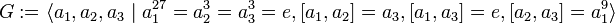# SmallGroup(243,19)

View a complete list of particular groups (this is a very huge list!)[SHOW MORE]

## Definition

This group of order$243 = 3^5$ is defined by means of the following presentation:$G := \langle a_1,a_2,a_3 \mid a_1^{27} = a_2^3 = a_3^3 = e, [a_1,a_2] = a_3, [a_1,a_3] = e, [a_2,a_3] = a_1^9 \rangle$

Here,$e$ denotes the identity element and$[ \ , \ ]$ denotes the commutator of two elements. Note that the left and right conventions for the commutator give different presentations but define isomorphic groups.

Want to compare and contrast arithmetic function values with other groups of the same order? Check out groups of order 243#Arithmetic functions

## GAP implementation

### Group ID

This finite group has order 243 and has ID 19 among the groups of order 243 in GAP's SmallGroup library. For context, there are groups of order 243. It can thus be defined using GAP's SmallGroup function as:

SmallGroup(243,19)

For instance, we can use the following assignment in GAP to create the group and name it$G$:

gap> G := SmallGroup(243,19);

Conversely, to check whether a given group$G$ is in fact the group we want, we can use GAP's IdGroup function:

IdGroup(G) = [243,19]

or just do:

IdGroup(G)

to have GAP output the group ID, that we can then compare to what we want.

### Description by presentation

gap> F := FreeGroup(3);;
gap> G :=F/[F.1^(27),F.2^3,F.3^3,Comm(F.1,F.2)*F.3^(-1),Comm(F.1,F.3),Comm(F.2,F.3)*F.1^(-9)];
<fp group on the generators [ f1, f2, f3 ]>
gap> IdGroup(G);
[ 243, 19 ]